Courses

# First Order Process Electrical Engineering (EE) Notes | EduRev

## Electrical Engineering (EE) : First Order Process Electrical Engineering (EE) Notes | EduRev

The document First Order Process Electrical Engineering (EE) Notes | EduRev is a part of Electrical Engineering (EE) category.
All you need of Electrical Engineering (EE) at this link: Electrical Engineering (EE)

First Order Process
A first order process is a process whose output y(t) is modeled by a first order differential equation.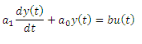35

where,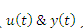are input and output of the process espectively. If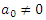, then define the following: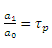and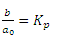Hence, the first order differential equation takes the following form: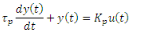36

At steady state condition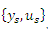, the equation can be re-written as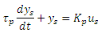37

Subtracting eq. (36) from eq. (37), we obtain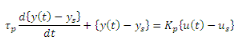38

Alternatively,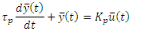39

Where,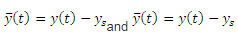are the deviation forms of the input and output variables of the process around the steady state, whose initial conditions are assumed to be the following: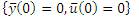.

Taking Laplace Transform of the eq. (39) we obtain,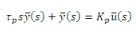40

Rearranging the above we obtain,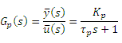41

Gp(s) is called the transfer function of the process. Kp and τp are called as gain and time constant of the process. The unit of gain is the ratio of the units of output to that of input, whereas the unit of time constant is same as that of time.

Example of a first order process

The following figure represents a water storage system.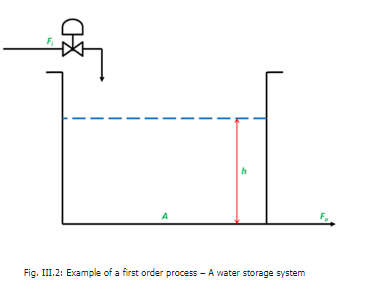It has a cylindrical tank of cross sectional area . Water flows into the tank with a rate of Fi and flows out of the tank with a rate of Fo . Height of water level is represented by h . A control valve, located on the inlet pipe, indicates that Fi can be considered as the manipulated input of the process whereas h can be considered as the controllable output of the process. From the basic knowledge of fluid mechanics (Bernoulli's principle), it is understood that pressure head of the water column provides the necessary kinetic energy for water to eject out of the tank at its bottom. Hence,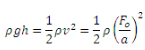42

where, ρ is the density of water, g is the gravitational constant, v is the velocity of water, a is the cross sectional area of the exit pipe. Thus,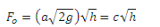43

Where, is a process constant. A simple material balance would yield the following equation:

Accumulation of water inside the tank = Water flow in – Water flow out

or,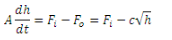44

The steady state (nominal) point of operation is around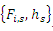. The above equation is a nonlinear equation and hence this needs to be linearized before a Laplace Transform can be used. Hence, in order to linearize the nonlinear term (√h) of the above equation around the nominal point of operation, Taylor series expansion is carried out on it as the following: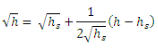45

Hence, eq.(44) can be re-written as,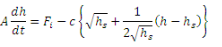46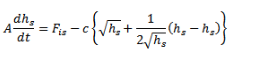47

Subtracting eq.(47) from eq. (46), we obtain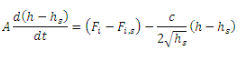48

or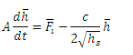49

Where,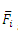and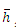are the deviation variables of inlet flow rate of water and height of the water level in the tank respectively. Taking the Laplace Transform of eq.(49), we obtain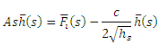50

or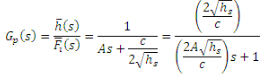51

The above equation indicates that the water storage system is a first order process whose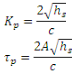52

Offer running on EduRev: Apply code STAYHOME200 to get INR 200 off on our premium plan EduRev Infinity!

,

,

,

,

,

,

,

,

,

,

,

,

,

,

,

,

,

,

,

,

,

;